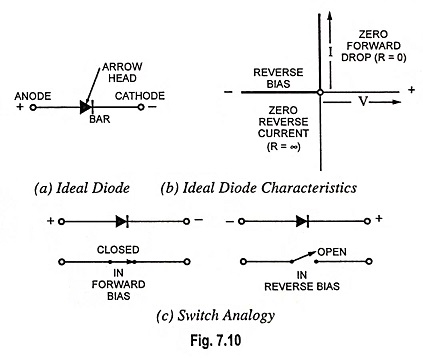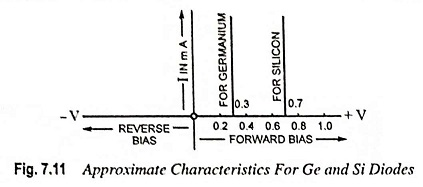## What is an Ideal Diode – Working and its V-I Characteristics:

Diode is a two terminal device that permits only unidirectional conduction. It conducts well in the forward direction and poorly in the reverse direction. It would have been ideal if it acted as a perfect conductor (with zero resistance or zero voltage drop across it) when forward biased and as a perfect insulator (with an infinite resistance or with no current through it) when reverse biased. The volt-ampere characteristics of such an ideal diode have been shown in Fig. 7.10 (b).An ideal diode acts like an automatic switch. The switch is closed when the diode is forward biased and is opened when reverse biased.

No diode can act as an ideal diode. An actual diode does not behave as a perfect conductor when forward biased and as a perfect insulator when reverse biased. Neither it offers zero resistance when forward biased nor infinite resistance when reverse biased. However, there are many applications in which diodes can he assumed to be nearly ideal devices, if the voltage drop across the diode when it is forward biased i.e., VD is taken into account. In cases when the circuit supply voltage Vs is much larger than the forward voltage drop VD, VD can be assumed constant without introducing any serious error.Also, the diode forward current I is usually so much larger than the reverse saturation current I0 that the I0 can just be ignored. These assumptions lead to a nearly ideal, or approximate, characteristics for germanium and silicon diodes, as illustrated in Fig. 7.11.

Scroll to Top